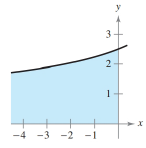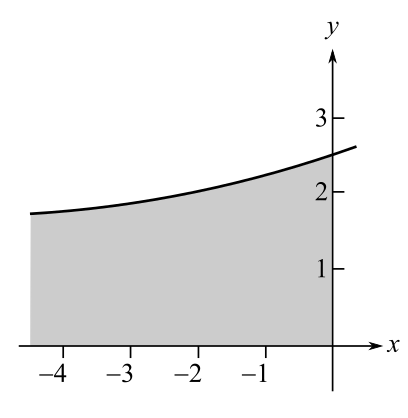Chapter 6.4, Problem 24E### Calculus: An Applied Approach (Min...

10th Edition
Ron Larson
ISBN: 9781305860919

#### Solutions

Chapter
Section### Calculus: An Applied Approach (Min...

10th Edition
Ron Larson
ISBN: 9781305860919
Textbook Problem
1 views

# Area of a Region In Exercises 21-26, find the area of the unbounded shaded region.To determine

To calculate: The area of shaded part for the provide graph:Explanation

Given Information:

The expression is provided as:

54xdx

Formula used:

From definition of improper integral.

bf(x)dx=limaabf(x)dx

Also, the expression for the integration of a polynomial is as follows:

xndx=xn+1n+1+C

Here, n0.

Calculation:

Consider the provided expression,

54xdx

For deciding the limit of integral, consider the provided graph,

From the provided graph, lower limit is and upper limit is 0 for the integration.

Use the property of improper integral and simplify as:

054xdx=limaa054xdx

Integrate the integrand by substation method as:

Assume 4x=u

Differentiate as:

dx=du

Now, substitute the values and integrate as:

### Still sussing out bartleby?

Check out a sample textbook solution.

See a sample solution

#### The Solution to Your Study Problems

Bartleby provides explanations to thousands of textbook problems written by our experts, many with advanced degrees!

Get Started

#### Find more solutions based on key concepts